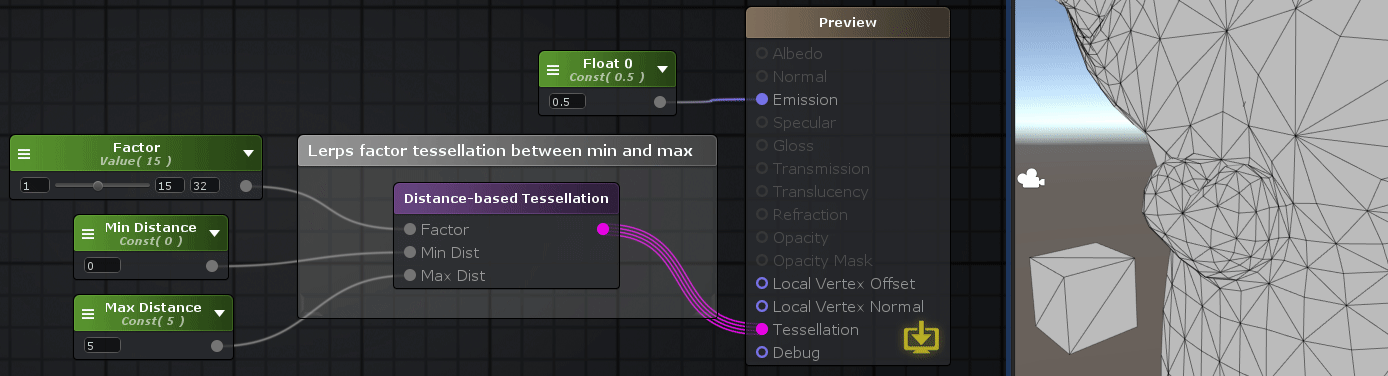# Unity Products:Amplify Shader Editor/Distance-based Tessellation

## Distance-based Tessellation Node

The Distance-based Tessellation Node sets up Tessellation according to the distance of the object using this shader to the camera via a Tessellation Factor, camera Minimum Distance and Maximum Distance . This technique allows the subdivision of a mesh's triangles, splitting them into smaller triangles at run-time in order to increase the surface detail of any given mesh. Tessellation will be highest near the minimum distance and lowest near the maximum distance. No tessellation will occur for distance values higher than Max Distance.

NOTE:Using this node is equivalent to activate the Tessellation option on the Master Node and set its Type to Distance-based. The Shader Model will be automatically set to 4.6 if its set to a lower value, since its the minimum version required.
Distance based tessellation should be used only if triangle sizes from the object mesh are similar. If not, situations may occur where small triangles may be too much tessellated and bigger ones are too little.
On these cases, Edge Length Tessellation should be used.Nodes used: Float, Distance-based Tessellation,

Node Parameter Description Default Value
Factor Tessellation Factor which determines the maximum amount tessellation/subdivisions that will be done. This value should be between [1-32].

Only visible if the respective input port is not connected.

0
Min Dist Minimum distance in meters to the camera where maximum tessellation should occur. Only visible if the respective input port is not connected. 0
Max Dist Maximum distance in meters to the camera where minimum tessellation should occur. Only visible if the respective input port is not connected. 0
Input Port Description Type
Factor Tessellation Factor which determines the maximum amount tessellation/subdivisions that will be done. This value should be between [1-32]. Float
Min Dist Minimum distance in meters to the camera where maximum tessellation should occur. Float
Max Dist Maximum distance in meters to the camera where minimum tessellation should occur. Float